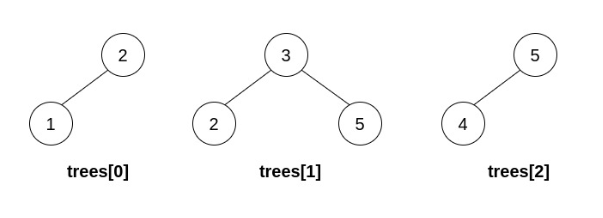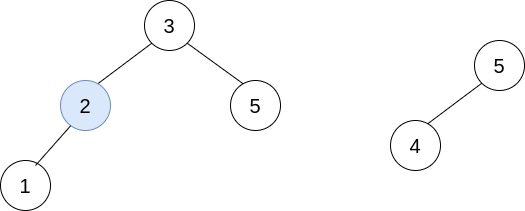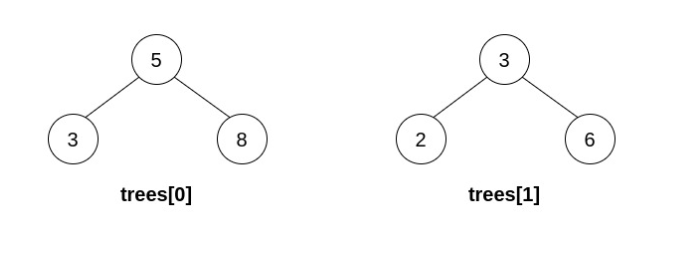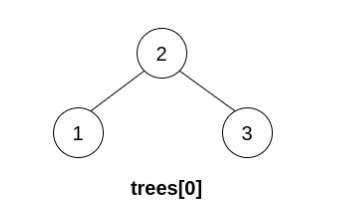Formatted question description: https://leetcode.ca/all/1932.html

# 1932. Merge BSTs to Create Single BST

Hard

## Description

You are given n BST (binary search tree) root nodes for n separate BSTs stored in an array trees (0-indexed). Each BST in trees has at most 3 nodes, and no two roots have the same value. In one operation, you can:

• Select two distinct indices i and j such that the value stored at one of the leaves of trees[i] is equal to the root value of trees[j].
• Replace the leaf node in trees[i] with trees[j].
• Remove trees[j] from trees.

Return the root of the resulting BST if it is possible to form a valid BST after performing n - 1 operations, or null if it is impossible to create a valid BST.

A BST (binary search tree) is a binary tree where each node satisfies the following property:

• Every node in the node’s left subtree has a value strictly less than the node’s value.
• Every node in the node’s right subtree has a value strictly greater than the node’s value.

A leaf is a node that has no children.

Example 1:Input: trees = [[2,1],[3,2,5],[5,4]]

Output: [3,2,5,1,null,4]

Explanation:

In the first operation, pick i=1 and j=0, and merge trees into trees.

Delete trees, so trees = [[3,2,5,1],[5,4]].In the second operation, pick i=0 and j=1, and merge trees into trees.

Delete trees, so trees = [[3,2,5,1,null,4]].The resulting tree, shown above, is a valid BST, so return its root.

Example 2:Input: trees = [[5,3,8],[3,2,6]]

Output: []

Explanation:

Pick i=0 and j=1 and merge trees into trees.

Delete trees, so trees = [[5,3,8,2,6]].The resulting tree is shown above. This is the only valid operation that can be performed, but the resulting tree is not a valid BST, so return null.

Example 3:Input: trees = [[5,4],]

Output: []

Explanation: It is impossible to perform any operations.

Example 4:Input: trees = [[2,1,3]]

Output: [2,1,3]

Explanation: There is only one tree, and it is already a valid BST, so return its root.

Constraints:

• n == trees.length
• 1 <= n <= 5 * 10^4
• The number of nodes in each tree is in the range [1, 3].
• No two roots of trees have the same value.
• All the trees in the input are valid BSTs.
• 1 <= TreeNode.val <= 5 * 10^4.

## Solution

Obviously, all leaf nodes except root nodes of all trees must have different values. Otherwise, there will be nodes with the same value in the merged tree, which is not a binary search tree. Therefore, loop over all trees and count the number of occurrences of all leaf nodes’ values. If there exists a value that occurs more than once, return null.

Use a hash map to store each root’s value and the corresponding root. Then for each leaf, if there exists a root with the same value as the leaf, replace the leaf with the root.

Finally, there should be only one tree remaining. Otherwise, return null. For the remaining tree, check whether it is a binary search tree, and return the tree if it is a binary search tree or null otherwise.

/**
* Definition for a binary tree node.
* public class TreeNode {
*     int val;
*     TreeNode left;
*     TreeNode right;
*     TreeNode() {}
*     TreeNode(int val) { this.val = val; }
*     TreeNode(int val, TreeNode left, TreeNode right) {
*         this.val = val;
*         this.left = left;
*         this.right = right;
*     }
* }
*/
class Solution {
public TreeNode canMerge(List<TreeNode> trees) {
Map<Integer, TreeNode> valRootMap = new HashMap<Integer, TreeNode>();
Map<TreeNode, int[]> minMaxMap = new HashMap<TreeNode, int[]>();
Map<TreeNode, int[]> legalRangeMap = new HashMap<TreeNode, int[]>();
for (TreeNode root : trees) {
valRootMap.put(root.val, root);
getMinMax(minMaxMap, root);
getLegalRange(legalRangeMap, root, Integer.MIN_VALUE, Integer.MAX_VALUE);
}
Map<Integer, Integer> leavesCountMap = new HashMap<Integer, Integer>();
for (TreeNode tree : trees)
visitLeaves(leavesCountMap, tree, true);
for (Map.Entry<Integer, Integer> entry : leavesCountMap.entrySet()) {
if (entry.getValue() > 1)
return null;
}
Set<TreeNode> set = new HashSet<TreeNode>(trees);
for (TreeNode root : trees) {
if (isLeaf(root))
continue;
TreeNode parent = getLeafParent(root);
TreeNode left = parent.left, right = parent.right;
if (left != null && valRootMap.containsKey(left.val)) {
int[] legalRange = legalRangeMap.get(left);
TreeNode prevRoot = valRootMap.get(left.val);
int[] minMax = minMaxMap.get(prevRoot);
if (minMax < legalRange || minMax > legalRange)
return null;
parent.left = prevRoot;
legalRangeMap.put(prevRoot, legalRange);
int[] parentMinMax = minMaxMap.get(parent);
int[] newMinMax = {minMax, parentMinMax};
minMaxMap.put(prevRoot, newMinMax);
set.remove(prevRoot);
}
if (right != null && valRootMap.containsKey(right.val)) {
int[] legalRange = legalRangeMap.get(right);
TreeNode prevRoot = valRootMap.get(right.val);
int[] minMax = minMaxMap.get(prevRoot);
if (minMax < legalRange || minMax > legalRange)
return null;
parent.right = prevRoot;
legalRangeMap.put(prevRoot, legalRange);
int[] parentMinMax = minMaxMap.get(parent);
int[] newMinMax = {parentMinMax, minMax};
minMaxMap.put(prevRoot, newMinMax);
set.remove(prevRoot);
}
}
if (set.size() != 1)
return null;
List<TreeNode> remain = new ArrayList<TreeNode>(set);
TreeNode root = remain.get(0);
if (isValidBST(root))
return root;
return null;
}

public void getMinMax(Map<TreeNode, int[]> minMaxMap, TreeNode root) {
TreeNode minNode = root, maxNode = root;
while (minNode.left != null)
minNode = minNode.left;
while (maxNode.right != null)
maxNode = maxNode.right;
minMaxMap.put(root, new int[]{minNode.val, maxNode.val});
}

public void getLegalRange(Map<TreeNode, int[]> legalRangeMap, TreeNode node, int min, int max) {
if (node == null)
return;
legalRangeMap.put(node, new int[]{min, max});
getLegalRange(legalRangeMap, node.left, min, node.val - 1);
getLegalRange(legalRangeMap, node.right, node.val + 1, max);
}

public void visitLeaves(Map<Integer, Integer> leavesCountMap, TreeNode node, boolean isRoot) {
if (isLeaf(node)) {
if (!isRoot)
leavesCountMap.put(node.val, leavesCountMap.getOrDefault(node.val, 0) + 1);
} else {
if (node.left != null)
visitLeaves(leavesCountMap, node.left, false);
if (node.right != null)
visitLeaves(leavesCountMap, node.right, false);
}
}

public boolean isLeaf(TreeNode node) {
return node != null && node.left == null && node.right == null;
}

public TreeNode getLeafParent(TreeNode root) {
if (root.left != null && root.right != null)
return root;
if (root.left != null) {
if (isLeaf(root.left))
return root;
else
return root.left;
} else {
if (isLeaf(root.right))
return root;
else
return root.right;
}
}

public boolean isValidBST(TreeNode root) {
if (root == null)
return true;
TreeNode prev = null;
boolean flag = false;
Deque<TreeNode> stack = new LinkedList<TreeNode>();
TreeNode node = root;
while (!stack.isEmpty() || node != null) {
while (node != null) {
stack.push(node);
node = node.left;
}
TreeNode curr = stack.pop();
if (prev != null) {
if (prev.val >= curr.val)
return false;
}
prev = curr;
node = curr.right;
}
return true;
}
}